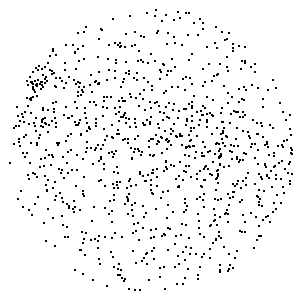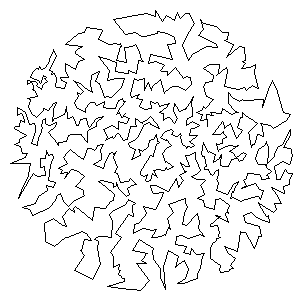COS 126 Traveling Salesperson Problem Programming Assignment Due: Wednesday, 11:59pm

Given a set of N points in the plane, the goal of a traveling salesperson is to visit all of them (and arrive back home) while keeping the total distance traveled as short as possible. Write a program to compute an approximate solution to the traveling salesperson problem (TSP), and use it to find the shortest tour that you can, connecting a given set of points in the plane.Perspective. The importance of the TSP does not arise from an overwhelming demand of salespeople to minimize their travel distance, but rather from a wealth of other applications, many of which seem to have nothing to do with the TSP at first glance. Real world application areas include: vehicle routing, circuit board drilling, VLSI design, robot control, X-ray crystallography, machine scheduling, and computational biology.

Greedy heuristics. The traveling salesperson problem is a notoriously difficult combinatorial optimization problem, In principle, one can enumerate all possible tours, but, in practice, the number of tours is so staggeringly large (roughly N factorial) that this approach is useless. For large N, no one knows an efficient method that can find the shortest possible tour for any given set of points. However, many methods have been studied that seem to work well in practice, even though they are not guaranteed to produce the best possible tour. Such methods are called heuristics. Your main task is to implement the nearest neighbor and smallest increase insertion heuristics for building a tour incrementally. Start with a one-point tour (from the first point back to itself), and iterate the following process until there are no points left.

• Nearest neighbor heuristic:  Read in the next point, and add it to the current tour after the point to which it is closest.

• Smallest increase heuristic:  Read in the next point, and add it to the current tour after the point where it results in the least possible increase in the tour length.

Point data type. First, create a Point data type that represents a point in the plane. Each Point object should be able to print itself to standard output, plot itself using StdDraw, plot a line segment from itself to another point, and calculate the Euclidean distance between itself and another point. It should have the following interface:

```public Point(double x, double y)    // constructor
public String toString()            // return string representation
public void draw()                  // draw point
public void drawTo(Point b)         // draw line from invoking point a to b
public double distanceTo(Point b)   // return Euclidean distance between the two points
```

Tour data type. Next, create a Tour data type that represents the sequence of points visited in a TSP tour. Represent the tour as a circular linked list of nodes, one for each point. Each Node will contain a Point and a reference to the next Node in the tour. Within Tour.java, define a nested class Node in the standard way.

```private class Node {
Point p;
Node next;
}```
Each Tour object should be able to print its constituent points to standard output, plot its points using StdDraw, compute its total distance, and insert a new point using either of the two heuristics. Your Tour data type should support the following interface:
```public Tour()                         // create an empty tour
public void show()                    // print the tour to standard output
public void draw()                    // draw the tour
public double distance()              // return the total distance of the tour
public void insertSmallest(Point p)   // insert p using the smallest insertion heuristic
public void insertNearest(Point p)    // insert p using the nearest neighbor heuristic
```

Input format. The input format will begin with two integers W and H, followed by pairs of real-valued x and y coordinates. All x coordinates will be betwen 0 and W; all y coordinates will be between 0 and H. As an example, the file tsp4.txt contains the following data

```600 600
532.6531 247.7551
93.0612 393.6735
565.5102 590.0000
10.0000  10.0000
```

Testing. Many test data files are also available. Once you implement Point and Tour, use the client program NearestInsertion.java to run the nearest insertion heuristic and print the resulting tour and its distance to standard output. Program SmallestInsertion.java is analogous but runs the smallest insertion heuristic. Programs NearestInsertionDraw.java and SmallestInsertionDraw.java are similar but they plot the results using StdDraw.

What to submit. Submit the files readme.txt, Tour.java, and Point.java.

Contest and extra credit. Implement a better heuristic. For example, observe that any tour with paths that cross can be transformed into a shorter one with no crossing paths: add that improvement to your program. Here are some other ideas. We will award a special prize to whomever finds the shortest tour around the 1000-point set.

This assignment was developed by Bob Sedgewick and Kevin Wayne.